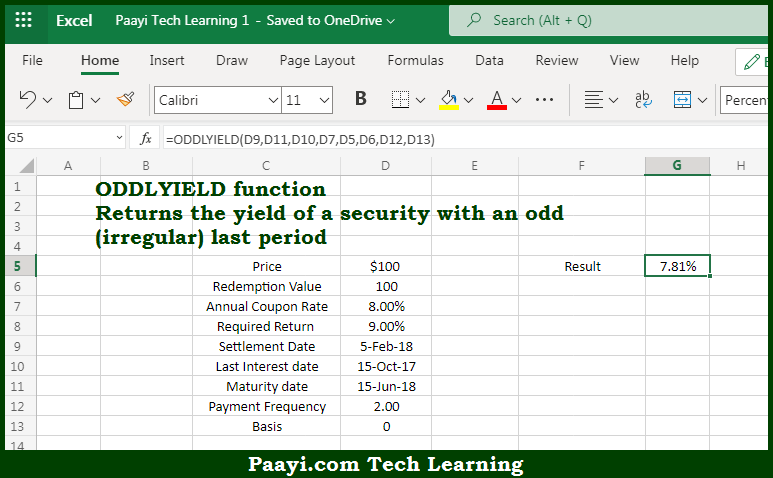# Learn How to Use Microsoft Excel ODDLYIELD Function

Written by | 0 Comments | 580 Views

In this article, you will learn how to use the Microsoft Excel ODDLYIELD function and its prime function in Microsoft Excel. You will also get to know the Microsoft Excel ODDLYIELD function return value and syntax with the help of some examples.

Microsoft Excel ODDLYIELD Function

The main purpose of the Microsoft Excel ODDLYIELD function is to get the yield of security where the last period will be odd. That implies, with the help of the ODDLYIELD function you can able to return the yield of security with an odd or irregular last period. So, with the help of the ODDLYIELD function, you can able to get the yield of security where the last period will be odd.

Return Value of ODDLYIELD Function

The return value will be the yield as a percentage.

Syntax of ODDLYIELD Function

=ODDLYIELD(sd, md, ld, rate, pr, redem, freq, [basis])

Where the arguments:

• sd - This is the settlement date of the security.
• md - This is the maturity date of the security.
• ld - This is the last interest date of the security.
• rate - This is the annual coupon rate of security.
• pr - This is the price per \$100 face value.
• redem - This is the redemption value per \$100 face value.
• freq - This is the coupon payments per year, where, annual = 1, semiannual = 2; quarterly = 4.
• basis - This is the day count basis, that's defaults to 0 (optional).

How to Use Microsoft Excel ODDLYIELD Function?So we know that Microsoft Excel ODDLYIELD function you can able to get get the yield of security where the last period will be odd. That implies, with the help of the ODDLYIELD function you can able to return the yield of security with an odd or irregular last period. So, with the help of the ODDLYIELD function, you can able to get the yield of security where the last period will be odd.